# Presse universelle MecaMotion par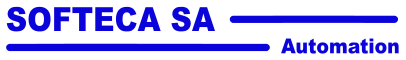Navigation: Part program instructions > Controls "Signal measurement" control instruction

"Signal measurement" control instruction

This instruction is used to measure the value of an "X" signal when a trigger signal "Y" reaches a certain threshold.

For a measurement of the "X" signal to be made, the trigger signal must be either larger or smaller (depending on the type of comparison) than the value of the threshold parameter.

When the signal "X" is measured, it is multiplied by a factor "A" and an offset "B" is added. This factor and offset are function parameters (by default, the factor is set to 1 and the offset is set to 0). These parameters can come from a user variable or be given as constants.

The result stored in the return variable will be equal to:

Resulting measured value  =  Measured value at threshold  *  Factor A  +  Offset B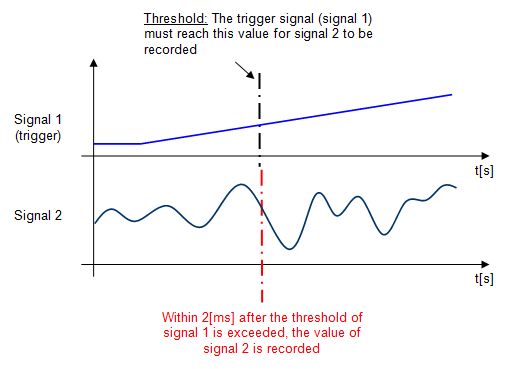Figure 1: Graph explaining how the "Signal Measurement" control works

The signals that can be defined as triggers are as follows:

Axis position

Force sensor

Measurement sensor signal

There are two types of trigger-to-threshold comparisons:

Comparison ">': If the trigger signal is greater than or equal to the threshold => Recording the value of the measured signal.

Comparison'<': If the trigger signal is smaller or equal to the threshold => Recording the value of the measured signal.

When the trigger signal passes the given threshold as a parameter, it is possible to record the value of one of the three signals below:

Axis position

Force sensor

Measurement sensor signal

Below is a list of the instruction's input and output parameters:

Parameter name

Declaration

Type of data

Default value

Description

Trigger signal

Input

Enum [Signal source]

Axis Position

Comparison trigger signal, measuring sensor, axis position or force sensor

Comparison

Input

Enum [Comparison]

>

Comparison type ">" or "<"

Threshold

Input

LREAL

0

Threshold that the trigger signal must reach to record the measured signal

Measured signal

Input

Enum [Signal source]

Force sensor

Measured signal, measuring sensor, axis position or force sensor

Factor

Input

LREAL

1

Factor multiplied by the result

Offset

Input

LREAL

0

Result

Output

LREAL

--

Measured value when the comparison becomes true * factor + offset

Table 1: List of instruction parameters

 Tip: If the "result" variable must contain the value that has actually been measured, simply set the following offset and multiplication parameters: Factor A = 1 Offset B = 0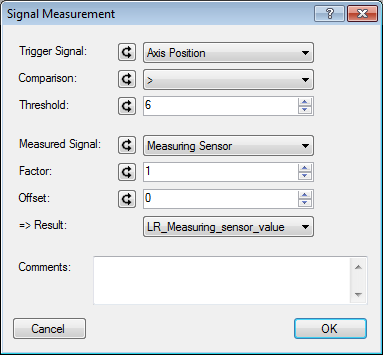Figure 2: Example of programming the signal measurement control

 Example of use: We would like to record the position of the axis at which the press begins to exert force on the part.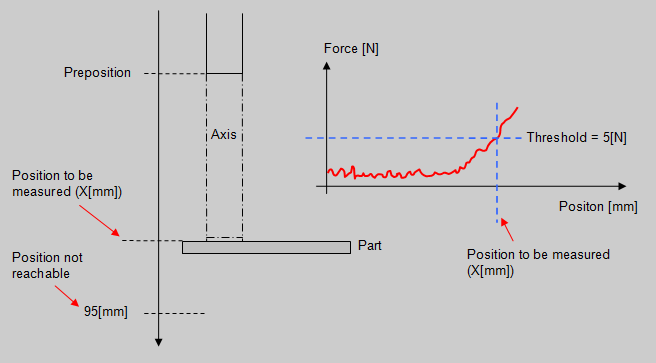Figure 3: Schematic explanation of the operation In the program below, we make a movement towards the preposition at high velocity, then a movement at low velocity to come into contact with the part (Force > 5[N]), at this moment we record the position of the axis and we stop the movement.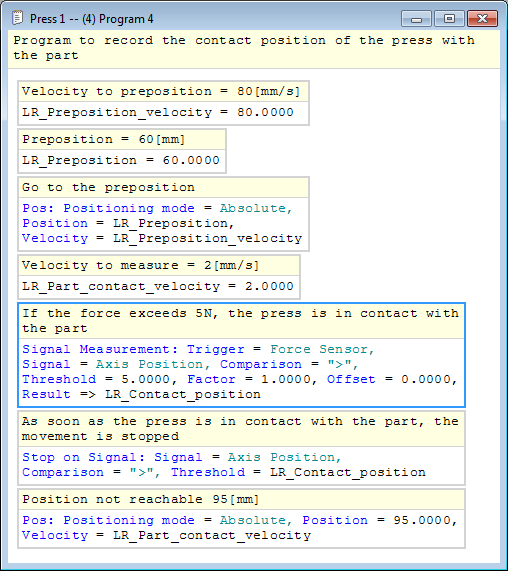Figure 4: Part program to record the position of the axis when the force > 5[N]